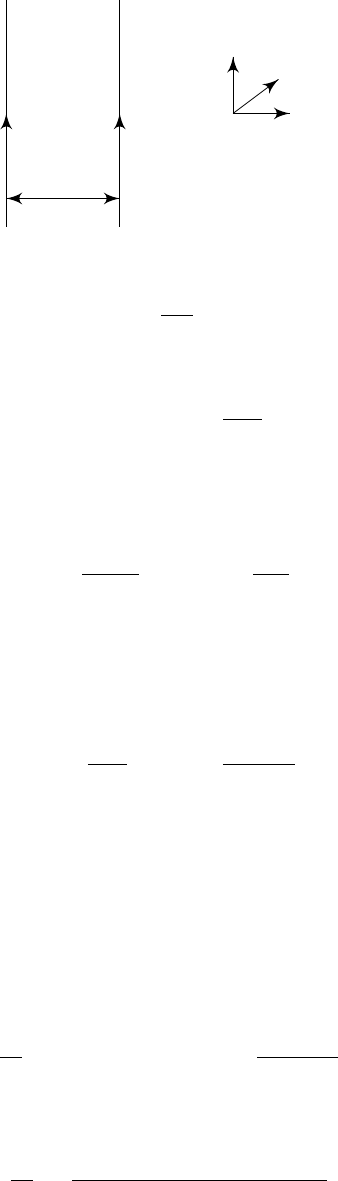3Magnetostatics

IB Electromagnetism3.4 Magnetic forces
We’ve seen that moving charge produces currents which generates a magnetic
field. But we also know that a charge moving in a magnetic field experiences a
force F = qr × B. So two currents will exert a force on each other.
Example (Two parallel wires).
d
z
x
y
We know that the field produced by each current is
B
1
=
µ
0
I
2πr
ˆϕ.
The particles on the second wire will feel a force
F = qv × B
1
= qv ×
µ
0
I
1
2πd
ˆ
y.
But
J
2
=
nqv
and
I
2
=
J
2
A
, where
n
is the density of particles and
A
is the
cross-sectional area of the wire. So the number of particles per unit length is
nA, and the force per unit length is
nAF =
µ
0
I
1
I
2
2πd
ˆ
z ×
ˆ
y = µ
0
I
1
I
2
2πd
ˆ
x.
So if
I
1
I
2
>
0, i.e. the currents are in the same direction, the force is attractive.
Otherwise the force is repulsive.
Example
(General force)
.
A current
J
1
, localized on some closed curve
C
1
, sets
up a magnetic field
B(r) =
µ
0
I
1
4π
I
C
1
dr
1
×
r r
1
|r r
1
|
3
.
A second current J
2
on C
2
experiences the Lorentz force
F =
Z
J
2
(r) × B(r) dV.
While we are integrating over all of space, the current is localized at a curve
C
2
.
So
F = I
2
I
C
2
dr
2
× B(r
2
).
Hence
F =
µ
0
4π
I
1
I
2
I
C
1
I
C
2
dr
2
×
dr
1
×
r
2
r
1
|r
2
r
1
|
3
.
For well-separated currents, approximated by
m
1
and
m
2
, we claim that the
force can be written as
F =
µ
0
4π
3(m
1
·
ˆ
r)(m
2
·
ˆ
r) (m
1
· m
2
)
r
3
,
whose proof is too complicated to be included.
Note that we’ve spent a whole chapter discussing “magnets”, but they are
nothing like what we stick on our fridges. It turns out that these “real” magnets
are composed of many tiny aligned microscopic dipoles arising from electron
spin. However, obviously we do not care about magnets in real life.